# Relational plots in Seaborn – Part II

Prerequisite: Relational Plots in Seaborn – Part I

In the previous part of this article, we learnt about the `relplot()`. Now, we will be reading about the other two relational plots, namely `scatterplot()` and `lineplot()` provided in seaborn library. Both these plots can also be drawn with the help of kind parameter in `relplot()`. Basically `relplot()`, by default, gives us scatterplot() only, and if we pass the parameter kind = “line”, it gives us `lineplot()`.

Example 1: Using relplot() to visualize tips dataset

 `import` `seaborn as sns ` `sns.``set``(style ``=``"ticks"``) ` `  `  `tips ``=` `sns.load_dataset(``'tips'``) ` `sns.relplot(x ``=``"total_bill"``, y ``=``"tip"``, data ``=` `tips) `

Output :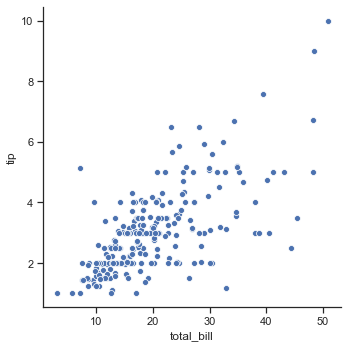Example 2: Using relplot() with kind=”scatter”.

 `import` `seaborn as sns ` ` `  ` `  `sns.``set``(style ``=``"ticks"``)  ` `tips ``=` `sns.load_dataset(``'tips'``) ` ` `  `sns.relplot(x ``=``"total_bill"``, ` `            ``y ``=``"tip"``, ` `            ``kind ``=``"scatter"``,  ` `            ``data ``=` `tips) `

Output :Example 3: Using relplot() with kind=”line”.

 `import` `seaborn as sns ` ` `  ` `  `sns.``set``(style ``=``"ticks"``)  ` `tips ``=` `sns.load_dataset(``'tips'``) ` ` `  `sns.relplot(x ``=``"total_bill"``, ` `            ``y ``=``"tip"``, ` `            ``kind ``=``"line"``, ` `            ``data ``=` `tips) `

Output :Though both these plots can be drawn using relplot(), seaborn also have separate functions for visualizing these kind of plots. These functions do provides some other functionalities too, compared to relplot(). Let us discuss about these function in more detail:

## Seaborn.scatterplot()

The scatter plot is a mainstay of statistical visualization. It depicts the joint distribution of two variables using a cloud of points, where each point represents an observation in the dataset. This depiction allows the eye to infer a substantial amount of information about whether there is any meaningful relationship between them.

Syntax :

`seaborn.scatterplot(x=None, y=None, data=None, **kwargs)`

Parameters :

Parameter Value Use
x, y numeric Input data variables
data Dataframe Dataset that is being used.
hue, size, style name in data; optional Grouping variable that will produce elements with different colors.
palette name, list, or dict; optional Colors to use for the different levels of the hue variable.
hue_order list; optional Specified order for the appearance of the hue variable levels.
hue_norm tuple or Normalize object; optional Normalization in data units for colormap applied to the hue variable when it is numeric.
sizes list, dict, or tuple; optional determines the size of each point in the plot.
size_order list; optional Specified order for appearance of the size variable levels
size_norm tuple or Normalize object; optional Normalization in data units for scaling plot objects when the size variable is numeric.
markers boolean, list, or dictionary; optional object determining the shape of marker for each data points.
style_order list; optional Specified order for appearance of the style variable levels
alpha float proportional opacity of the points.
legend “brief”, “full”, or False; optional If “brief”, numeric hue and size variables will be represented with a sample of evenly spaced values. If “full”, every group will get an entry in the legend. If False, no legend data is added and no legend is drawn.
ax matplotlib axes; optional Axes object in which the plot is to be drawn.
kwargs key, value pairings Other keyword arguments are passed through to the underlying plotting function.

Example 1: Plotting a scatterplot using marker to differentiate between timing of the people visiting the restaurant.

 `import` `seaborn as sns ` ` `  ` `  `sns.``set``(style ``=``"ticks"``)  ` `tips ``=` `sns.load_dataset(``'tips'``) ` `markers ``=` `{``"Lunch"``: ``"s"``, ``"Dinner"``: ``"X"``} ` ` `  `ax ``=` `sns.scatterplot(x ``=``"total_bill"``, ` `                     ``y ``=``"tip"``,  ` `                     ``style ``=``"time"``, ` `                     ``markers ``=` `markers, ` `                     ``data ``=` `tips) `

Output: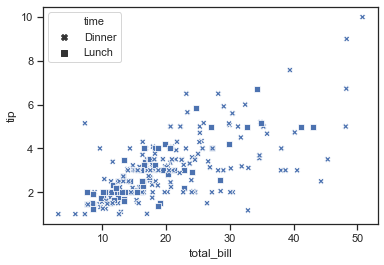Example 2: Passing data vectors instead of names in a data frame.

 `import` `seaborn as sns ` ` `  ` `  `iris ``=` `sns.load_dataset(``"iris"``) ` ` `  `sns.scatterplot(x ``=` `iris.sepal_length, ` `                ``y ``=` `iris.sepal_width, ` `                ``hue ``=` `iris.species, ` `                ``style ``=` `iris.species) `

Output: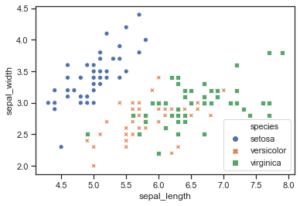## Seaborn.lineplot()

Scatter plots are highly effective, but there is no universally optimal type of visualization. For certain datasets, you may want to consider changes as a function of time in one variable, or as a similarly continuous variable. In this case, drawing a line-plot is a better option.

Syntax :

`seaborn.lineplot(x=None, y=None, data=None, **kwargs)`

Parameters :

Parameter Value Use
x, y numeric Input data variables
data Dataframe Dataset that is being used.
hue, size, style name in data; optional Grouping variable that will produce elements with different colors.
palette name, list, or dict; optional Colors to use for the different levels of the hue variable.
hue_order list; optional Specified order for the appearance of the hue variable levels.
hue_norm tuple or Normalize object; optional Normalization in data units for colormap applied to the hue variable when it is numeric.
sizes list, dict, or tuple; optional determines the size of each point in the plot.
size_order list; optional Specified order for appearance of the size variable levels
size_norm tuple or Normalize object; optional Normalization in data units for scaling plot objects when the size variable is numeric.
markers, dashes boolean, list, or dictionary; optional object determining the shape of marker for each data points.
style_order list; optional Specified order for appearance of the style variable levels
units long_form_var Grouping variable identifying sampling units. When used, a separate line with correct terminology will be drawn for each unit but no legend entry will be inserted. Useful for displaying experimental replicate distribution when exact identities are not necessary.
estimator name of pandas method or callable or None; optional Method for aggregating the vector y at the same x point through multiple observations. If None, all observations will be drawn.
ci int or “sd” or None; optional Size of the confidence interval to be drawn when aggregating with an estimator. “sd” means drawing a standard deviation.
n_boot int; optional> Number of bootstraps to use for confidence interval measurement.
seed int, numpy.random.Generator, or numpy.random.RandomState; optional Seed or random number generator for reproducible bootstrapping.
sort bool; optional is True, sorts the data.
err_style “band” or “bars”; optional Either using translucent error bands to display the confidence intervals or discrete error bars.
err_kws dict of keyword arguments Additional paramters to control the aesthetics of the error bars.
legend “brief”, “full”, or False; optional If “brief”, numeric hue and size variables will be represented with a sample of evenly spaced values. If “full”, every group will get an entry in the legend. If False, no legend data is added and no legend is drawn.
ax matplotlib axes; optional Axes object in which the plot is to be drawn.
kwargs key, value pairings Other keyword arguments are passed through to the underlying plotting function.

Example 1: Basic visualization of “fmri” dataset using lineplot()

 `import` `seaborn as sns ` ` `  ` `  `sns.``set``(style ``=` `'whitegrid'``) ` `fmri ``=` `sns.load_dataset(``"fmri"``) ` ` `  `sns.lineplot(x ``=``"timepoint"``, ` `             ``y ``=``"signal"``, ` `             ``data ``=` `fmri) `

Output :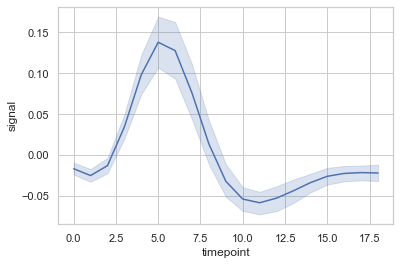Example 2: Grouping data points on the basis of category, here as region and event.

 `import` `seaborn as sns ` ` `  ` `  `sns.``set``(style ``=` `'whitegrid'``) ` `fmri ``=` `sns.load_dataset(``"fmri"``) ` ` `  `sns.lineplot(x ``=``"timepoint"``, ` `             ``y ``=``"signal"``, ` `             ``hue ``=``"region"``, ` `             ``style ``=``"event"``, ` `             ``data ``=` `fmri) `

Output :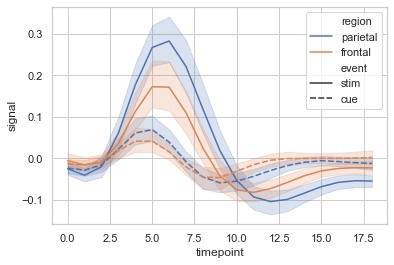Example 3: A complex plot visualizing “dots” dataset, to show the power of seaborn. Here, in this example quantitative color mapping is used.

 `import` `seaborn as sns ` ` `  ` `  `sns.``set``(style ``=` `'whitegrid'``) ` `dots ``=` `sns.load_dataset(``"dots"``).query(``"align == 'dots'"``) ` ` `  `sns.lineplot(x ``=``"time"``,  ` `             ``y ``=``"firing_rate"``, ` `             ``hue ``=``"coherence"``, ` `             ``style ``=``"choice"``, ` `             ``data ``=` `dots) `

Output :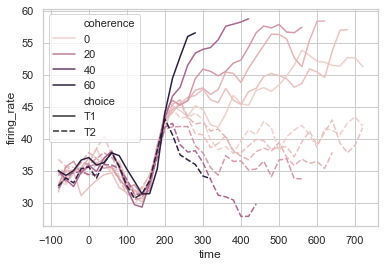Attention geek! Strengthen your foundations with the Python Programming Foundation Course and learn the basics.

To begin with, your interview preparations Enhance your Data Structures concepts with the Python DS Course.

My Personal Notes arrow_drop_upCheck out this Author's contributed articles.

If you like GeeksforGeeks and would like to contribute, you can also write an article using contribute.geeksforgeeks.org or mail your article to contribute@geeksforgeeks.org. See your article appearing on the GeeksforGeeks main page and help other Geeks.

Please Improve this article if you find anything incorrect by clicking on the "Improve Article" button below.

Article Tags :

Be the First to upvote.

Please write to us at contribute@geeksforgeeks.org to report any issue with the above content.